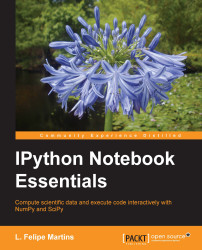•#### IPython Notebook Essentials#### Overview of this book

IPython Notebook EssentialsCreditswww.PacktPub.comPrefaceFree Chapter
A Tour of the IPython NotebookThe Notebook InterfaceGraphics with matplotlibHandling Data with pandasAdvanced Computing with SciPy, Numba, and NumbaProIPython Notebook Reference CardA Brief Review of PythonNumPy ArraysIndex## Chapter 3. Graphics with matplotlib

This chapter explores matplotlib, an IPython library for production of publication-quality graphs. In this chapter, the following topics will be discussed:

• Two-dimensional plots using the `plot` function and setting up line widths, colors, and styles

• Plot configuration and annotation

• Three-dimensional plots

• Animations

Being an IPython library, matplotlib consists of a hierarchy of classes, and it is possible to code using it in the usual object-oriented style. However, matplotlib also supports an interactive mode. In this mode, the graphs are constructed step-by-step, thus adding and configuring each component at a time. We lay emphasis on the second approach since it is designed for the rapid production of graphs. The object-oriented style will be explained whenever it is needed or leads to better results.

### Note

The sense in which the word interactive is used in this context is somewhat different from what is understood today. Graphs produced by matplotlib are...